# Simulating Pharmacokinetic Concentration-Time Profiles With the linpk Package

## Introduction

If you work in pharmacometrics, you have probably at some time or another written code to generate concentrations from a PK model by implementing the closed-form solution to a simple system of linear differential equations. The mathematical equations are readily available in many textbooks or online (for instance, Mathematical Expressions of the Pharmacokinetic and Pharmacodynamic Models implemented in the Monolix software by Bertrand and Mentré).

Each formula is specific with regard to:

• Dosing type: bolus, infusion (zero-order), absorption (first-order)
• Number of doses: single, multiple, at steady state
• Number of compartments: 1, 2, 3, …

Implementing a different function for each combination you are likely to encounter is cumbersome, time consuming and error prone. Enter the linpk package, which conveniently uses a single function, pkprofile, to handle all combinations of the above.

Meanwhile, there are now powerful and efficient ODE solvers in R that make it easy to simulate from any PK model, including mrgsolve, PKPDsim, and RxODE. These packages are much more powerful than linpk, which can only solve linear systems of ODE. What linpk offers in return is convenience; most models can be simulated from using a single line of code, and there is no need to write out the model in differential equation form or perform a compilation step. In addition, linpk has built-in convenience functions to calculate secondary PK parameters, such as half-life, AUC or Cmax, from the simulated concentration-time profile.

## Getting started

The first example will be deliberately simple, a 1-compartment model and a single IV bolus dose. This will serve as a starting point to see how more complex models and dosing can be accommodated.

First, we will create a vector of times at which to generate concentrations. In this example it will be a fine grid of equally-spaced time points from 0 to 24 hours:

t.obs <- seq(0, 24, 0.1)

(A note on units: here we are assuming here that time is hours, but we are free to use any time unit we like, as long as the units for clearances and rate constants correspond. For instance, if time is in days, and volume in L, than clearance is in L/day).

Next, we use pkprofile to generate the concentrations at those times following a 100 mg IV bolus dose, and assuming a clearance of 0.5 L/h and a (central) volume of 11 L:

y <- pkprofile(t.obs, cl=0.5, vc=11, dose=list(amt=100))
plot(y)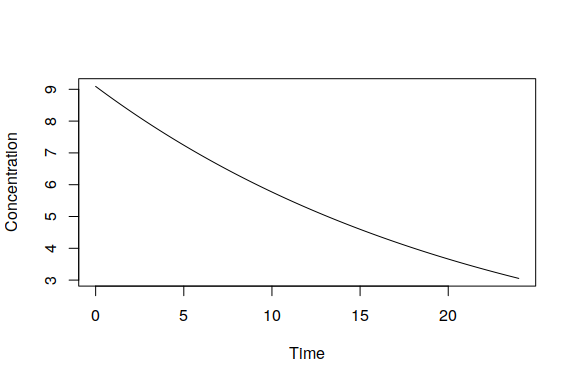There are a few things to note here. One is that the dose argument is a list; it can also be a data.frame (which is a special kind of list).

The object y is a simple object that can essentially be treated as a numeric vector of concentrations at the times t.obs, but it also has a few methods for convenience (for instance, it can be plotted). The result can also be converted to a data.frame, with columns time and conc by default:

sim <- as.data.frame(y)
tail(sim)
##     time     conc
## 236 23.5 3.123934
## 237 23.6 3.109766
## 238 23.7 3.095663
## 239 23.8 3.081624
## 240 23.9 3.067648
## 241 24.0 3.053736

## Dosing type

To change from an IV bolus to an infusion, we simply add either the dur (duration) or rate of the infusion to the dose list argument. For example, to simulate the same 100 mg dose but infused over 90 minutes, we would do:

y <- pkprofile(t.obs, cl=0.5, vc=11, dose=list(amt=100, dur=1.5))
plot(y)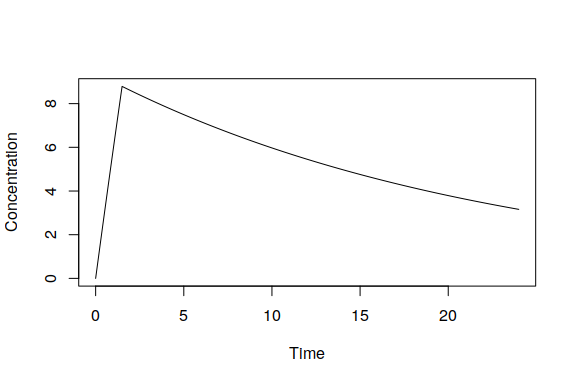To simulate an oral dose (i.e., a dose administered to a depot compartment with first-order absorption), we simply specify the absorption rate constant ka:

y <- pkprofile(t.obs, cl=0.5, vc=11, ka=1.3, dose=list(amt=100))
plot(y)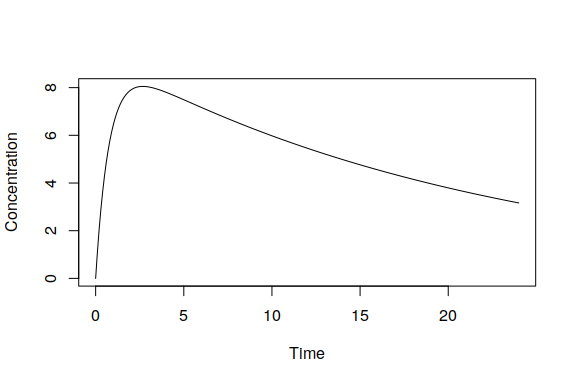The function recognizes by the presence of this parameter that a first-order absorption is required. Note that if both dur (or rate) and ka are specified, then the function will deduce that the dose is infused into the depot compartment.

A dose may have an associated time lag and bioavailability (f) (note that lag and f are associated to a dose, not to a compartment as in NONMEM). These parameters can be added to the dose list argument as necessary:

y <- pkprofile(t.obs, cl=0.5, vc=11, ka=1.3, dose=list(amt=100, lag=0.4, f=0.6))
plot(y)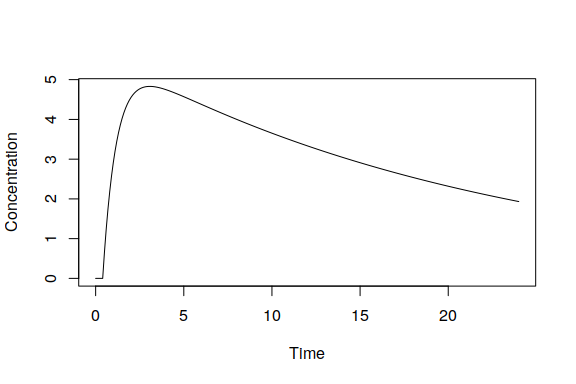## Multiple doses

There are two ways to specify multiple doses to pkprofile. The first is to add dose times to the dose list argument:

y <- pkprofile(t.obs, cl=0.5, vc=11, ka=1.3, dose=list(t.dose=c(0, 12), amt=100))
plot(y)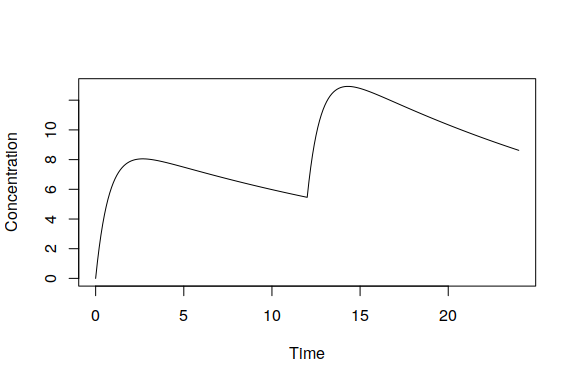In this example, we have simulated two doses, one given at time zero, and the second at 12 hours. Note that vector recycling has been applied to amt to match the number of doses (this is generally true for items in the dose list; shorter items are recycled to match the longest item in the list). We can also specify different amounts for each dose:

y <- pkprofile(t.obs, cl=0.5, vc=11, ka=1.3, dose=list(t.dose=c(0, 12), amt=c(100, 50)))
plot(y)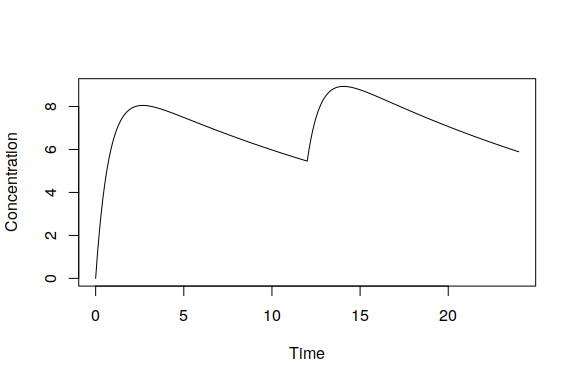It is also possible to use a data.frame (which is a type of list) to specify doses:

doses <- data.frame(t.dose=c(0, 12), amt=c(100, 50))
doses
##   t.dose amt
## 1      0 100
## 2     12  50
y <- pkprofile(t.obs, cl=0.5, vc=11, ka=1.3, dose=doses)
plot(y)The second way to specify multiple doses is to use the addl argument for additional doses, along with the inter-dose interval ii. Thus, to simulate regular doses of 100 mg every 12 hours for 5 days, we could do the following:

t.obs <- seq(0, 6*24, 0.5)
y <- pkprofile(t.obs, cl=0.5, vc=11, ka=1.3, dose=list(amt=100, addl=9, ii=12))
plot(y)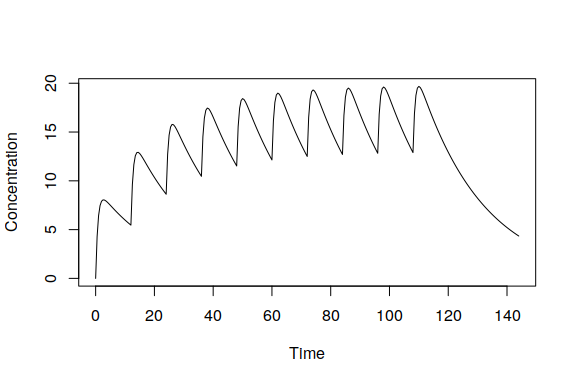A different way of achieving the same, without using addl, is to use seq() to specify a vector of dose times:

y <- pkprofile(t.obs, cl=0.5, vc=11, ka=1.3, dose=list(t.dose=seq(0, 9*12, 12), amt=100))
plot(y, col="red")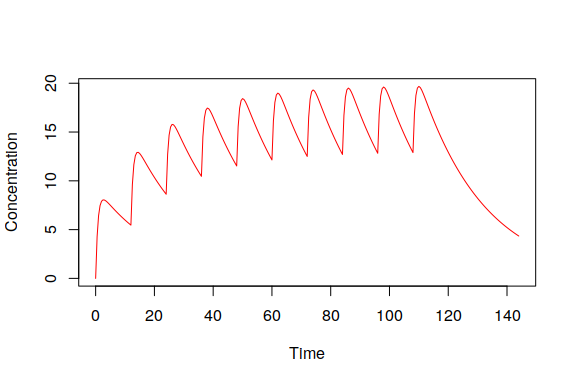We can, of course, mix the different options as well. To specify 100 mg doses every 24 hours, with an extra dose of 50 mg at 14 hours on day 3, we can do the following:

y <- pkprofile(t.obs, cl=0.5, vc=11, ka=1.3,
dose=list(t.dose=c(0, 24*2 + 14), amt=c(100, 50), addl=c(4, 0), ii=24))
plot(y)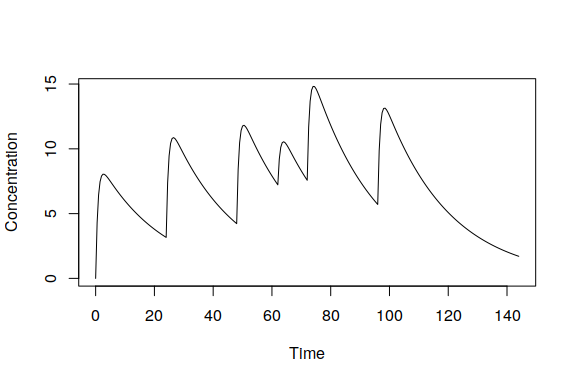A data.frame with all the realized doses can also obtained using the dose.frame function:

dose.frame(y)
##     t.dose amt rate dur ii    ss cmt lag f     conc
## 1        0 100    0   0 24 FALSE   0   0 1 0.000000
## 1.1     24 100    0   0 24 FALSE   0   0 1 3.164379
## 1.2     48 100    0   0 24 FALSE   0   0 1 4.227328
## 2       62  50    0   0 24 FALSE   0   0 1 7.222502
## 1.3     72 100    0   0 24 FALSE   0   0 1 7.574075
## 1.4     96 100    0   0 24 FALSE   0   0 1 5.708597

One can specify a dose given at steady state by adding ss=T or ss=1 to the dose list argument. Going back to a previous examples, we had regular regular doses of 100 mg every 12 hours for 5 days. To see that steady state has been reached after 5 days, let us overlay the steady state profile in green:

t.obs <- seq(0, 6*24, 0.5)
y <- pkprofile(t.obs, cl=0.5, vc=11, ka=1.3, dose=list(amt=100, addl=9, ii=12))
plot(y)
yss <- pkprofile(t.obs, cl=0.5, vc=11, ka=1.3, dose=list(amt=100, addl=9, ii=12, ss=T))
lines(yss, col="green3")
legend("bottomright", c("Steady state"), col=c("green3"), lty=1, bty="n")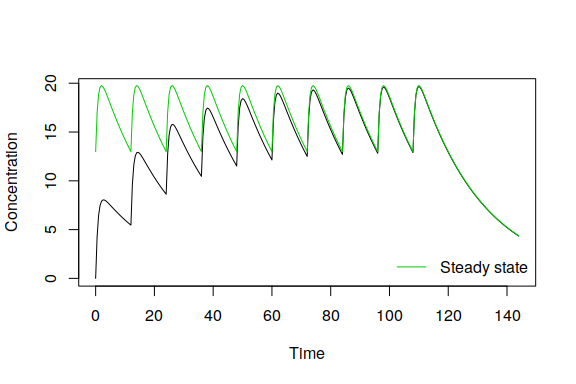## Number of compartments

The pkprofile function makes it easy to specify models with 1, 2, 3 or more compartments (practically speaking, you will never go over 3 compartments, but the function is not restricted). The way to specify the number of compartments is to include the parameters that are appropriate to the model. The mandatory parameters are cl and vc. For a 2-compartment model, add q and vp:

t.obs <- seq(0, 24, 0.1)
y2 <- pkprofile(t.obs, cl=0.5, vc=11, q=2, vp=30, ka=1.3, dose=list(amt=100))

For a 3 (or more) compartments, you can specify q and vp as vectors:

y3 <- pkprofile(t.obs, cl=0.5, vc=11, q=c(2, 0.3), vp=c(30, 3), ka=1.3, dose=list(amt=100))
plot(y2)
lines(y3, col="green3")
legend("topright", c("2-Compartment", "3-Compartment"), col=c("black", "green3"), lty=1, bty="n")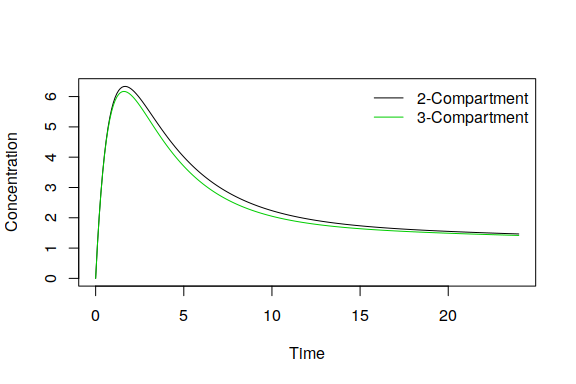## Secondary parameters

### Half-lives

linpk makes it quite easy to access some secondary parameters from the PK model and simulation. The half-lives of the model can be obtained directly using the halflife() function. For instance for the 3-compartment model with first-order absorption in the previous section:

y <- pkprofile(t.obs, cl=0.5, vc=11, q=c(2, 0.3), vp=c(30, 3), ka=1.3, dose=list(amt=100))
halflife(y)
##       HL.1       HL.2       HL.3       HL.a
##  2.1946882  7.2720612 68.8588820  0.5331901

There are 4 components: the $$\alpha$$, $$\beta$$ and $$\gamma$$ half-lives, as well as the absorption half-life.

Note that computing the half-life doesn’t require any doses or sampling. For example, this 2-compartment model:

halflife(pkprofile(cl=0.5, vc=11, q=2, vp=30))
##      HL.1      HL.2
##  2.447202 64.788075

### Other secondary parameters (Cmin, Cmax, AUC, …)

Other secondary parameters are accessed using the secondary() function:

secondary(y)
##   From To   N Ctrough Cmin     Cmax     Cave      AUC Tmin Tmax
## 1    0 24 241       0    0 6.170103 2.512857 60.30857    0  1.6

Note that these secondary parameters are computed based on the simulated profile, so the time points specified in t.obs are important and relevant. This function optionally takes arguments From and To to specify intervals over which the parameters are computed. Looking at an example with multiple dosing, we observe that when not specified, the default is to compute secondary parameters over the intervals defined by the dose times:

t.obs <- seq(0, 5*24, 0.1)
y <- pkprofile(t.obs, cl=0.5, vc=11, q=2, vp=30, ka=1.3, dose=list(amt=100, addl=5, ii=24))
plot(y)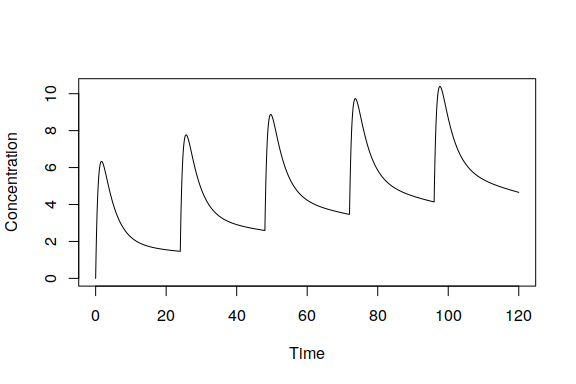secondary(y)
##   From  To   N  Ctrough     Cmin      Cmax     Cave       AUC Tmin Tmax
## 1    0  24 241 0.000000 0.000000  6.335438 2.660562  63.85349    0  1.7
## 2   24  48 241 1.466404 1.466404  7.771659 3.946255  94.71012   24 25.7
## 3   48  72 241 2.592773 2.592773  8.877722 4.939629 118.55110   48 49.7
## 4   72  96 241 3.464063 3.464063  9.733716 5.708050 136.99319   72 73.6
## 5   96 120 241 4.138048 4.138048 10.396262 6.302459 151.25902   96 97.6

But we can easily ask for the AUC from 0 to 12 hours, 0 to 48 hours, and 48 to 128 hours, for instance:

secondary(y, From=c(0, 0, 48), To=c(12, 48, 128))
##   From  To   N  Ctrough     Cmin      Cmax     Cave       AUC Tmin Tmax
## 1    0  12 121 0.000000 0.000000  6.335438 3.679664  44.15597    0  1.7
## 2    0  48 481 0.000000 0.000000  7.771659 3.303409 158.56361    0 25.7
## 3   48 120 721 2.592773 2.592773 10.396262 5.650046 406.80331   48 97.6

## Combining IV infusion with first-order absorption

It is possible to specify the compartment that a particular dose is administered to using the cmt element of the dosing list argument. The central compartment is always associated with the number 1, while specifying cmt=0 indicates that the dose goes to the default dosing compartment, which is the depot compartment in the presence of ka (first-order absorption), and the central compartment otherwise. This feature is used in the following example to simulate a scenario in which a 150 mg IV dose is administered at time zero, followed by daily subcutaneous 10 mg doses (first-order absorption) on days 8 to 20 (here we will assume that the unit of time is days so clearance is in L/day). In this example, we specify the dose list as an external data.frame:

t.obs <- seq(0, 20, 0.1)
doses <- data.frame(t.dose=c(0, 7), amt=c(150, 10), addl=c(0, 12), ii=1, cmt=c(1, 0), dur=c(1/24, 0))
doses
##   t.dose amt addl ii cmt        dur
## 1      0 150    0  1   1 0.04166667
## 2      7  10   12  1   0 0.00000000
y <- pkprofile(t.obs, cl=0.8, vc=6, q=0.09, vp=4.5, ka=1.3, dose=doses)
plot(y, col="blue", main="150 mg IV at time zero, 10 mg SC QD on days 8 to 20", xlab="Time (days)")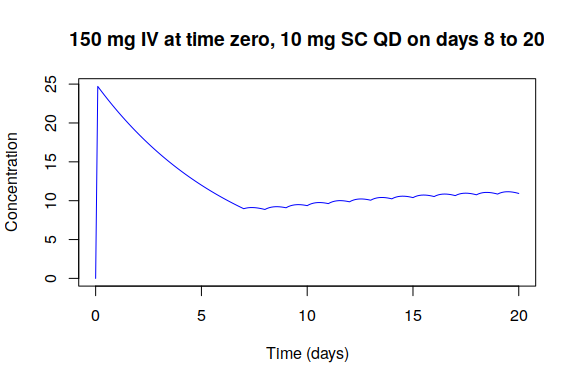## Time-varying parameters

Time-varying parameters are not handled directly. Systems with parameters that vary continuously over time are not linear and hence outside the scope of linpk. However, if parameters change at a discrete set of time points, and the system is linear between those time points, it is possible to advance the system in discrete steps. To do so, we can use the object returned by pkprofile as the first argument in a subsequent call in order to append to the existing profile. For example, the following simulates a scenario with daily oral dosing where clearance increases by 20% each day:

t.grid <- seq(0, 24, 0.1)
t.dose <- seq(0, by=24, len=8)
cl0 <- 0.1; cl <- cl0; ka <- 0.5; vc <- 10
y <- pkprofile(t.obs=t.grid, cl=cl, vc=vc, ka=ka, dose=list(t.dose=0))
for (day in 2:8) {
cl <- 1.2*cl
y <- pkprofile(y, t.obs=t.grid + t.dose[day], cl=cl, vc=vc, ka=ka, dose=list(t.dose=t.dose[day]))
}
plot(y, main="Clearance increasing by 20% each day")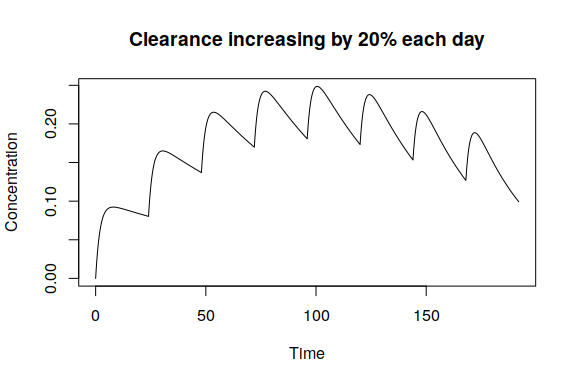Caution: This approach does NOT work if the parameters change in the middle of a zero-order infusion. In that case, it would be necessary to split the infusion at the time when the parameters change.

## Shiny app

The package includes a small shiny app for interactive exploration. To run it locally, the following packages must be installed: shiny, shinyjs, shinyAce, dygraphs. It can then be run by the command:

linpk::linpkApp()

The app will open in a browser, and looks like this: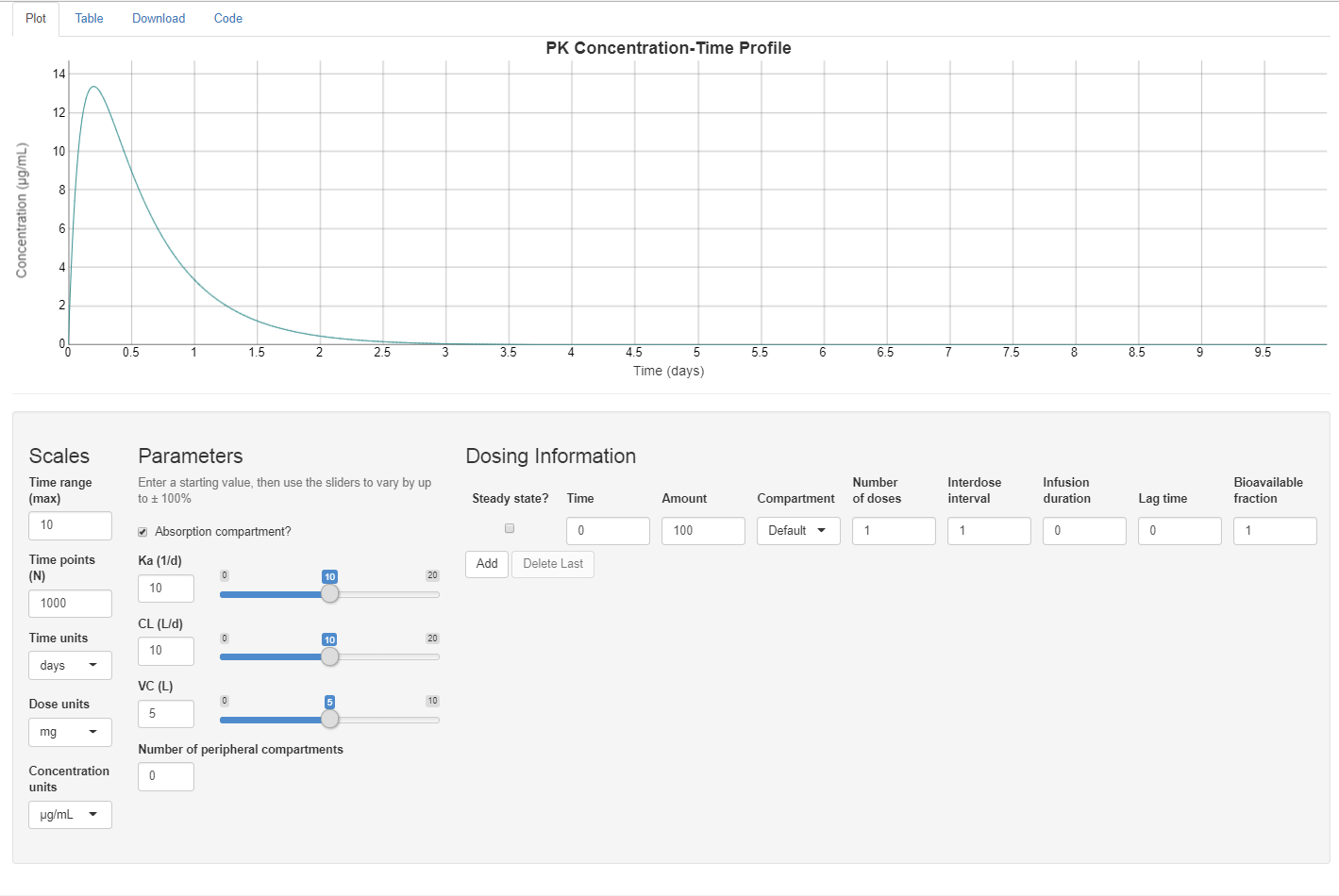Screenshot of the shiny app

You can enter different doses, vary the PK parameters by number or slider, and modify the units and time scale. There are tabs along the top to show a table of derived secondary parameters, to download a CSV file of the simulated data points, and to generate source code for the simulation that can be copied directly to a script.

## Limitations

Compared to more general ODE solvers (mrgsolve, PKPDsim, RxODE), this package has some limitations, the most obvious being that it can only simulate from linear PK models. This means that it cannot be used to simulate from most PD models, for instance. Another disadvantage is that simulating time-varying parameters must be done with iterative function calls.